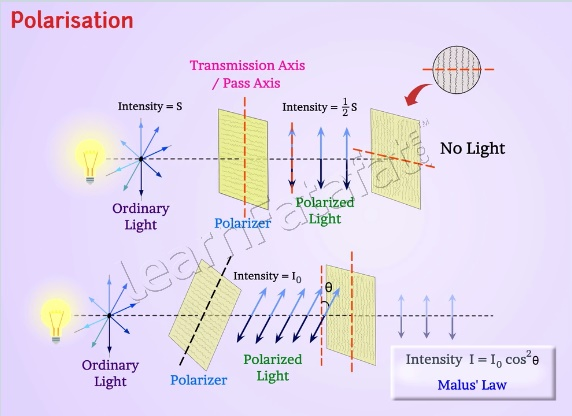# Class 12 Physics Chapter 10 Wave Optics

During summers, brightness is unbearable to the eye. When you wear sunglasses, intensity of light gets reduced and eyes feel better. The phenomena behind this is, polarization. In this article, you will get detailed understanding of polarization of light.

### Polarization of light

Light travels in random oscillations of electric field, perpendicular to the direction of propagation. It means that displacement is randomly changing with time. Such light is called unpolarised light. For such electric fields vector can take all possible direction in the plane, perpendicular to direction of light propagation randomly and rapidly. If it is possible to restrict the vibrations in any one plane then such light is called polarized light.Polarization

There are various methods to produce plane polarised light from unpolarised light. The instrument that produces polarised light from unpolarised light is called polariser. Polaroid is a plane sheet containing thin plastic like sheet, made of long chains of molecules aligned in a particular direction. When ordinary light fall perpendicular to the sheet, the electric field parallel to the chains get absorbed so as to set up current in the chains, however, the field perpendicular to chains get pass through. The direction perpendicular to the direction of the chains is called transmission axis or pass axis of the polaroid. However, the light coming out of the polaroid have electric field vector, parallel to transmission axis. It is interesting to know that intensity of polarised light reduces to half of ordinary light.

However, if this polarised light is allowed to fall on the second polaroid such that the electric field is parallel to transmission axis then the light is completely passed through it. Moreover if the electric field is perpendicular to the transmission axis then the light is completely stopped by the polaroid. If the electric field at some angle θ with the transmission axis, only part of light is transmitted, the intensity of light for angle θ can be calculated by I = I0 cos2 θ . Here, I0is the intensity of polarized light after passing through first polaroid. This expression is also known as as Malus Law. Polaroids are used to control the intensity, in sunglasses, window panes, etc. These are also used in photographic cameras and 3D movie cameras.

### Polarization by scatteringPolarization due to Scattering

Light is polarized by scattering. Just for an example, watch the clear blue portion of sky using a polaroid, by rotating it to different angles, there is rise and fall of intensity. It is part of light that have changes its direction after scattering from atmospheric molecule. However, light is transverse in character, all direction of vibrations of electric vector in unpolarised light are confined to single plane, perpendicular to wave motion. Because of components vibrating in plane, when the light wave fall on the molecule it causes, vibration of charges in the plane perpendicular to propagation of wave. During vibration, these charges radiate energy.
Consider electrons vibrating in vertical and horizontal directions. We know that accelerating electrons generate electromagnetic wave, here, it is light wave. Thus, vibration of electrons in both directions radiate energy towards observer, if observation is done at any angle other than 90°. Here, light is partially polarised. In case, if observation is done at any angle other than 90°. Here light is partially polarised. If observation is done at 90°, then the charges accelerating in vertical direction does not radiate energy towards observer and only the charges accelerating in horizontal direction radiates energy towards observer. Light observed is restricted in plane, so light is polarised light.

### Polarization by reflection

Consider light is incident on transparent medium having refractive index n. If angle of incidence is 0° or 90°, the light waves remains unpolarised. If light is incident at some other angle then part of light is reflected and part of light is refracted. Unpolarised light has two electric field components, perpendicular to plane of incidence represented by dots and other, in the plane of incidence. At angle of incidence i, when reflected light and refracted light is perpendicular to each other, the reflected light is polarised with the electric field components perpendicular to plane of incidence. However, refracted ray is never completely polarised. The angle of incidence in such case is called as Brewster’s angle denoted by iB.
From Snell’s law,
μ = sin tB / sin r = sin tB / sin( π/2 – tB ) = sin tB / cos tB= tan tB
Thus, tan iB = n. This is known as Brewster’s Law

Keywords: Polarisation, Snell’s law, Brewster’s Law, Malus Law, Refraction, Reflection, scattering of light, Unpolarised light

Share Fatafat
•
•
•
•
•